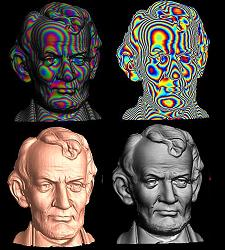Optimal Mass Transportation Map Code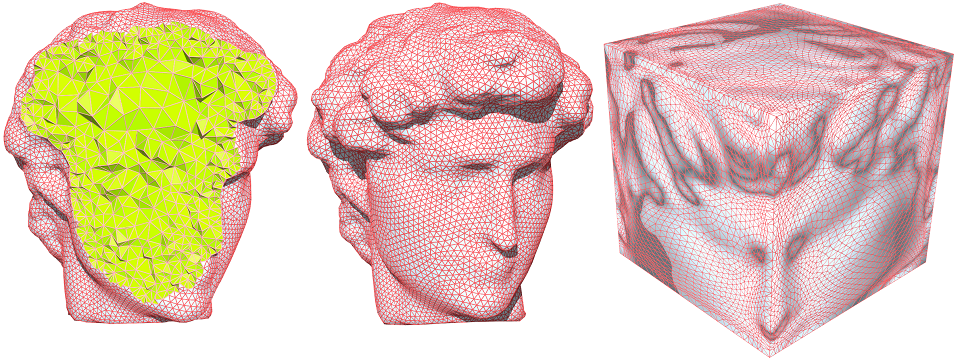This tutorial introduces the concept and the theorem of optimal mass transportation map, with computational algorithms. The code and the data sets can be found here: [HTML]

Tutorial on Harmonic Map with C++ source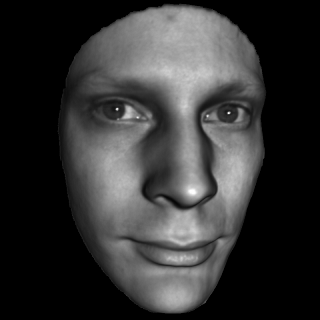This tutorial introduces the concept and the theorem of surface harmonic map, with computational algorithms. The source code and the data sets can be found here: [HTML]

Tutorial on Mesh Viewer with C++ source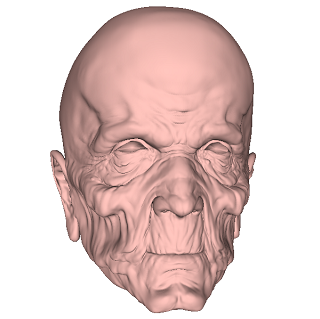This tutorial introduces a simple triangle mesh viewer using OpenGL and Glut. The source code and the data sets can be found here: [HTML]

Tutorial on Surface Ricci Flow with C++ source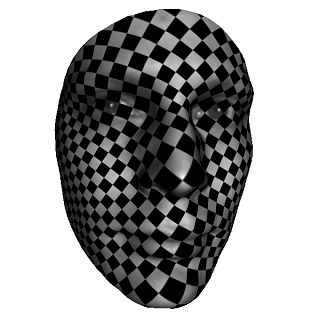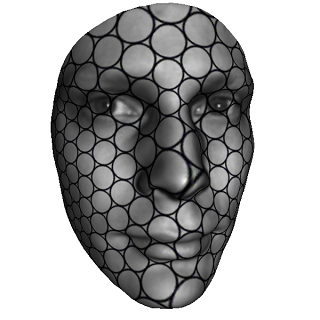This tutorial introduces the discrete surface Ricci flow method with concepts, algorithm. The source code and the data sets can be found here: [HTML]

Tutorial on Riemann with C++ source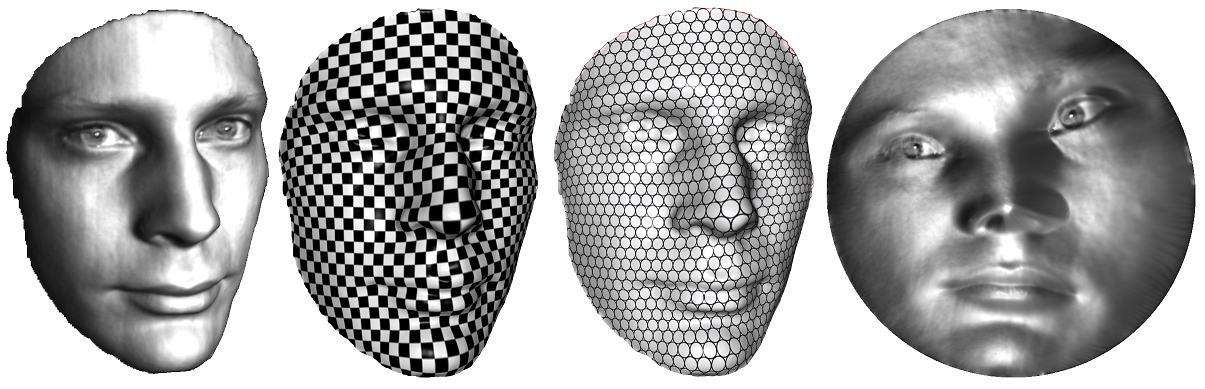This tutorial introduces the surface Riemann mapping method with concepts, algorithm. The source code and the data sets can be found here: [HTML]RiemannMapper: A General Purpose Mesh Parameterization Tool Kit

A general purpose mesh parameterization tool kit, with sample mesh data with different topologies. The binary code can be downloaded from here.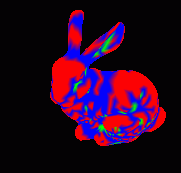(source code)

A general purpose mesh library with source code. It is easy to transport it to SGI unix, linux and windows, download from here.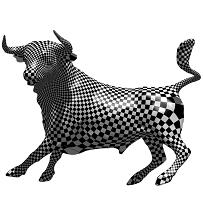Computational Conformal Geometry Arachive